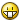# Get Array From Array (opposite _ArrayDelete)

Go to solution Solved by mikell,

## Recommended Posts

Hello

I need Get Array From Array

_ArrayDelete working fine on this code

```#include <array.au3>
#include <Excel.au3>

Global \$oExcel = _Excel_Open()
Global \$oWorkbook = _Excel_BookOpen(_Excel_Open(), @ScriptDir&"\E.xlsx")
If @error Then Exit MsgBox(\$MB_SYSTEMMODAL, "", "Error opening E.xlsx")

\$1 = \$aExcel

\$Find = _ArrayFindAll(\$aExcel, "2", Default, Default, Default, Default,5)
_ArrayInsert(\$Find, 0, UBound(\$Find))
_ArrayDelete(\$aExcel, \$Find)
\$2 = \$aExcel

_ArrayDisplay(\$1)
_ArrayDisplay(\$2)```

But i need get All Rows containing the value 2 on Column 5

difference between two arrays

Tnx.

Edited by ahmeddzcom
##### Share on other sites

Try  this if ok

```#include <array.au3>
#include <Excel.au3>

Global \$oExcel = _Excel_Open()
Global \$oWorkbook = _Excel_BookOpen(_Excel_Open(), @ScriptDir&"\E.xlsx")
If @error Then Exit MsgBox(\$MB_SYSTEMMODAL, "", "Error opening E.xlsx")

_ArrayDisplay(\$aExcel)

Local \$aResult = _ArrayFindAll(\$aExcel, 2, Default, Default, Default, Default, 5)
_ArrayDisplay(\$aResult, "Found in Column 5")```

##### Share on other sites

12 minutes ago, ioa747 said:

Try  this if ok

i need difference between two arrays 😄

##### Share on other sites

I don't understand, the difference is that you deleted it or you're just looking for a way to confirm it?

##### Share on other sites

22 minutes ago, ioa747 said:

I don't understand, the difference is that you deleted it or you're just looking for a way to confirm it?

i need get All Rows containing the value 2 on Column 5

Edited by ahmeddzcom
##### Share on other sites

and what it gives you this?

```#include <array.au3>
#include <Excel.au3>

Global \$oExcel = _Excel_Open()
Global \$oWorkbook = _Excel_BookOpen(_Excel_Open(), @ScriptDir&"\E.xlsx")
If @error Then Exit MsgBox(\$MB_SYSTEMMODAL, "", "Error opening E.xlsx")

;~         _ArrayDisplay(\$aExcel)

Local \$aResult = _ArrayFindAll(\$aExcel, 2, Default, Default, Default, Default, 5)
_ArrayDisplay(\$aResult, "Found in Column 5")```

##### Share on other sites

or in comparison

```#include <array.au3>
#include <Excel.au3>

Global \$oExcel = _Excel_Open()
Global \$oWorkbook = _Excel_BookOpen(_Excel_Open(), @ScriptDir & "\E.xlsx")
If @error Then Exit MsgBox(\$MB_SYSTEMMODAL, "", "Error opening E.xlsx")

\$1 = \$aExcel

\$Find = _ArrayFindAll(\$aExcel, "2", Default, Default, Default, Default, 5)
_ArrayInsert(\$Find, 0, UBound(\$Find))
_ArrayDelete(\$aExcel, \$Find)
\$2 = \$aExcel

;~         _ArrayDisplay(\$1, "\$1")
;~         _ArrayDisplay(\$2, "\$2")

For \$x = 0 To \$1[UBound(\$1) - 1]
ConsoleWrite(\$1[\$x] & "=" & _GetID(\$1[\$x]) & @CRLF)
Next

Func _GetID(\$RowID)
Local \$ID
For \$i = 0 To \$1[UBound(\$2) - 1]
\$ID = 0
If \$RowID = \$2[\$i] Then
\$ID = \$2[\$i]
ExitLoop
EndIf
Next
Return \$ID
EndFunc   ;==>_GetID```

Edited by ioa747
##### Share on other sites

I just noticed that \$i = 0 is wrong because \$RowID starts with 0.

So it's better this way

```#include <array.au3>
#include <Excel.au3>

Global \$oExcel = _Excel_Open()
Global \$oWorkbook = _Excel_BookOpen(_Excel_Open(), @ScriptDir & "\E.xlsx")
If @error Then Exit MsgBox(\$MB_SYSTEMMODAL, "", "Error opening E.xlsx")

\$1 = \$aExcel

\$Find = _ArrayFindAll(\$aExcel, "2", Default, Default, Default, Default, 5)
_ArrayInsert(\$Find, 0, UBound(\$Find))
_ArrayDelete(\$aExcel, \$Find)
\$2 = \$aExcel

;~         _ArrayDisplay(\$1, "\$1")
;~         _ArrayDisplay(\$2, "\$2")

For \$x = 0 To \$1[UBound(\$1) - 1]
ConsoleWrite(\$1[\$x] & "=" & _GetID(\$1[\$x]) & @CRLF)
Next

Func _GetID(\$RowID)
Local \$ID
For \$i = 0 To \$1[UBound(\$2) - 1]
\$ID = "REMOVED"
If \$RowID = \$2[\$i] Then
\$ID = \$2[\$i]
ExitLoop
EndIf
Next
Return \$ID
EndFunc   ;==>_GetID```

##### Share on other sites

I think OP would like to easily extract some non-contiguous full rows from an array, when the row indices are indicated in an external 1D array. For example something like this, using... a non-existant function :

`Local \$aExtract = _ArrayExtractEx(\$aExcel, \$aIndices)`

IIRC Melba23 added this functionality to _ArrayDelete (and _ArrayInsert too ?), I mean the possibility of using a range of indices in a 1D array. By the way, OP used this functionality when he scripted :

`_ArrayDelete(\$aExcel, \$Find)`

\$Find being a 1D array of indices, returned by _ArrayFindAll

Of course it should be easy for OP to extract these full rows in a loop, based on the \$Find array, but it would be easier with a one liner.

But wait... maybe it's already possible and I missed it, everything is possible##### Share on other sites

?

```#include <array.au3>
#include <Excel.au3>

Global \$oExcel = _Excel_Open(False)
Global \$oWorkbook = _Excel_BookOpen(\$oExcel, @ScriptDir&"\E.xlsx", False, False)
If @error Then Exit MsgBox(\$MB_SYSTEMMODAL, "", "Error opening E.xlsx")

\$value = "2"
\$a = StringRegExp(_ArrayToString(_Excel_RangeRead(\$oWorkbook)), '(?m)^(?:[^\|]*\|){5}' & \$value & '\|.*\$', 3)
Local \$res
For \$i = 0 to UBound(\$a)-1
Next
_ArrayDisplay(\$res)```##### Share on other sites

40 minutes ago, mikell said:

?

```#include <array.au3>
#include <Excel.au3>

Global \$oExcel = _Excel_Open(False)
Global \$oWorkbook = _Excel_BookOpen(\$oExcel, @ScriptDir&"\E.xlsx", False, False)
If @error Then Exit MsgBox(\$MB_SYSTEMMODAL, "", "Error opening E.xlsx")

\$value = "2"
\$a = StringRegExp(_ArrayToString(_Excel_RangeRead(\$oWorkbook)), '(?m)^(?:[^\|]*\|){5}' & \$value & '\|.*\$', 3)
Local \$res
For \$i = 0 to UBound(\$a)-1
Next
_ArrayDisplay(\$res)```Nice Work Mr.@mikell

Can do this without StringRegExp ?

##### Share on other sites

7 hours ago, pixelsearch said:

But wait... maybe it's already possible and I missed it, everything is possibleExact.

But i can't do this. 😔

##### Share on other sites

• Solution
4 hours ago, ahmeddzcom said:

Can do this without StringRegExp ?

Of course you canOnce the \$aExcel array is converted to a string then you can use StringSplit, details below

```#include <array.au3>
#include <Excel.au3>

Global \$oExcel = _Excel_Open(False)
Global \$oWorkbook = _Excel_BookOpen(\$oExcel, @ScriptDir&"\E.xlsx", False, False)
If @error Then Exit MsgBox(\$MB_SYSTEMMODAL, "", "Error opening E.xlsx")

Local \$value = "2", \$col = 5
Local \$res[UBound(\$aExcel, 2)]
\$txt = _ArrayToString(\$aExcel)

#cs
\$a = StringRegExp(\$txt, '(?m)^(?:[^\|]*\|){' & \$col & '}' & \$value & '\|.*\$', 3)
For \$i = 0 to UBound(\$a)-1
Next
#ce

\$rows = StringSplit(\$txt, @crlf, 1)
For \$i = 1 to \$rows
\$cells = StringSplit(\$rows[\$i], "|", 2)
If \$cells[\$col] = \$value Then _ArrayAdd(\$res, \$rows[\$i])
Next
_ArrayDisplay(\$res)```

Edited by mikell

## Create an account

Register a new account

• ### Recently Browsing   0 members

×

• Wiki

• Back

• #### Beta

• Git
• FAQ
×
• Create New...